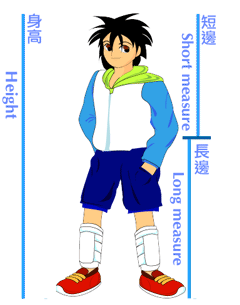## Belly Button Ratios

Measure your height in inches and divide it into two measures.1. The first measures the length from your feet to your belly button.

Use the formulas below to find your belly button ratios.

Long Measure - The distance from your belly button to the bottom of your foot.

First Ratio = height/long measure = __________
Second Ratio = long measure/short measure = __________

Put your ratios on the board.

1. Find the average of the first ratios: __________
2. Find the average of the second ratios: __________

## Part Two: Fibobelly Ratios:

1. Calculate 1/1: __________
2. Calculate 2/1: __________
3. Calculate 3/2: __________
4. Calculate 5/3: __________
5. Calculate 8/5: __________
6. Calculate 13/8: __________
7. Calculate 21/13: __________
8. Calculate 34/21: __________
9. Calculate 55/34: __________
10. Calculate 89/55: __________
11. Calculate 144/89: __________
12. Calculate 233/144: __________
13. Calculate (1+√5) ÷ 2 ____________________
14. Calculate 1 ÷ 1.618033988 ____________________

Are either of the ratios in 3 or 4 close to the Fibonacci ratio above?